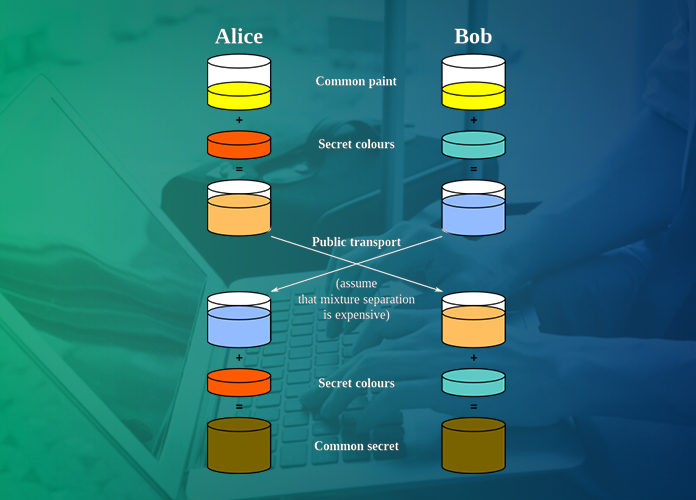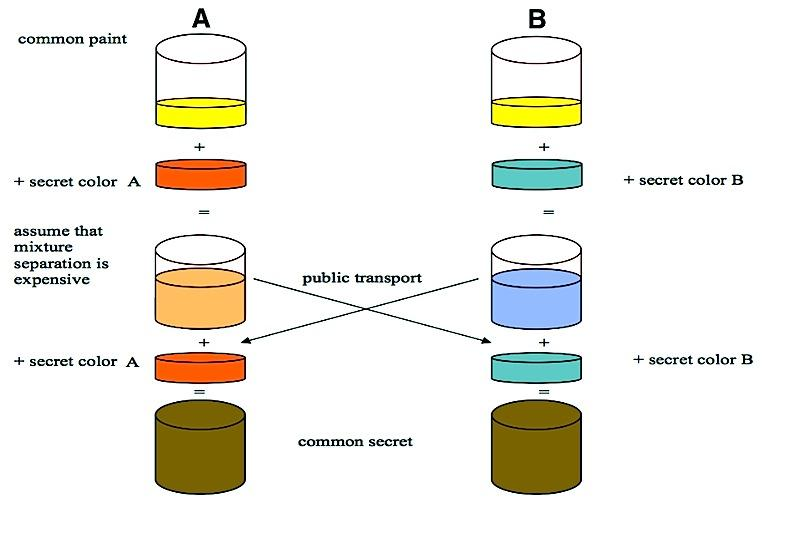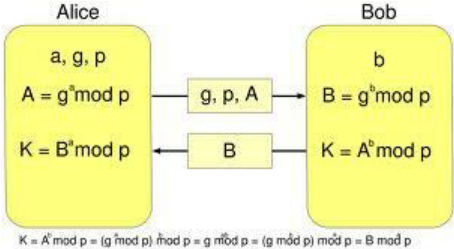# What Is Diffie Hellman Key Exchange?

0
2169The key exchange problem is an important and well-studied problem in the area of cryptography and information security. The problem is regarding how two parties (assumed to have no prior information about each other that could be leveraged to create a key) can exchange cryptographic keys between them over an insecure channel such that no third-party can obtain a copy. The exchanged keys can then be used for encryption and decryption of further information exchanges between the two parties. It is imperative to secure the manner of the key exchange otherwise any third-party who can oversee the exchange process can effectively access any subsequent encrypted information exchanges between the two parties. The Diffie Hellman Key Exchange is a secure key exchange protocol widely used in practical cryptographic applications.

## The Diffie Hellman Key Exchange

In 1974, Whitfield Diffie and Martin Hellman proposed a scheme for secure exchange of keys over an insecure channel. It is a public key distribution scheme, which means instead of a single key, a pair of keys is used, one of which is channeled publicly. It was one of the first public key distribution protocols and is still widely used in practice today.

The General Scheme

The Diffie Hellman can be easily understood by using colors as an aid rather than numbers. We’ll first look at the general idea behind the scheme and then delve into its mathematical details.

Say A and B are the two parties in the exchange. The objective is to come up with a shared secret that can be used as the basis of a key without explicitly communicating the key. The scheme works as follows:

• Both of them initially agree upon a starting color. Note that the choice of the color is public and anyone can see what color they chose.A comes up with a secret color of his own and doesn’t disclose this color to anyone (not even to B). He then mixes this color to the original color and sends the mixture to B. B does the same thing (mixes his own secret color to the original color) and sends it to A. These mixtures are also transmitted publicly, but it is highly impractical to discern the added secret colors from the mixtures (this is the crucial part of the process).
A and B, upon receiving the mixtures from their counterparts, add their own secret colors to the mixtures, thereby ending up with the same color. This color is now a shared secret and can form the basis of the cryptographic key.

The following picture1 illustrates this process:Note that any third party that can oversee the exchange has only access to the initial mixtures and the original color. Since the secret colors cannot be made known using these, the final mixture of colors remains known only to A and B.

Mathematical Implementation

The Diffie Hellman exchange relies on the inability of modern computational resources to solve what is called the Diffie Hellman Problem. The problem is stated as:
Given the values of two integers of the form and , what is the value of ?

Although the problem appears simple, it is notoriously difficult to solve. The fastest way of solving the problem is to solve the Discrete Logarithm Problem (which asks for the discrete logarithm of a given number to a given base), which is well studied and has been proven to be unsolvable in polynomial time. Consequently, there are no known algorithms that can solve this problem in a reasonable amount of time using current computational resources (although an efficient algorithm was developed by Peter Shor, it uses quantum computation techniques which haven’t been realized yet). Leveraging this, the key exchange protocol is formulated. It is outlined as follows (Alice and Bob are the assumed participants):

• Alice begins the exchange by coming up with numbers , , and . is Alice’s private key, and are chosen such that is a primitive root modulo. Alice then calculates A = , which is Alice’s public key, and sends , and A to Bob via the insecure channel.
Bob also comes up with his own private key b. Bob then receives g , p and A, and calculates B = , and K= . He then sends B, which is Bob’s public key, to Alice through the channel.
Alice receives B and calculates mod . Note that this value equals K, because = = = K (since is primitive root modulo ).
Alice and Bob now share the common value K, which can now be used as an encryption key for all further communication.

The following figure1 illustrates this process:This implementation is secure because any third-party has access only to ,g, p, A and B. Since the Diffie Hellman Problem is intractable; for suitable choices of and , it is almost impossible to discern a and b, and hence impossible to determine K. The scheme also owes it success to the fact that although a and b cannot be computed efficiently; computing , B and K is efficient using what is called modular exponentiation. Hence, the key exchange takes an insignificant amount of time to complete.

It is important to note that although the Diffie-Hellman scheme provides an effective way to exchange keys, it doesn’t provide authentication of any kind. That is to say, neither of the two parties participating in the exchange can be completely certain that they are indeed communicating with the other intended party instead of some other third-party. Authentication is a crucial part of any information exchange, since the security provided by the encryption using the shared key is rendered ineffective if the key exchange itself takes place with a malicious third-party.